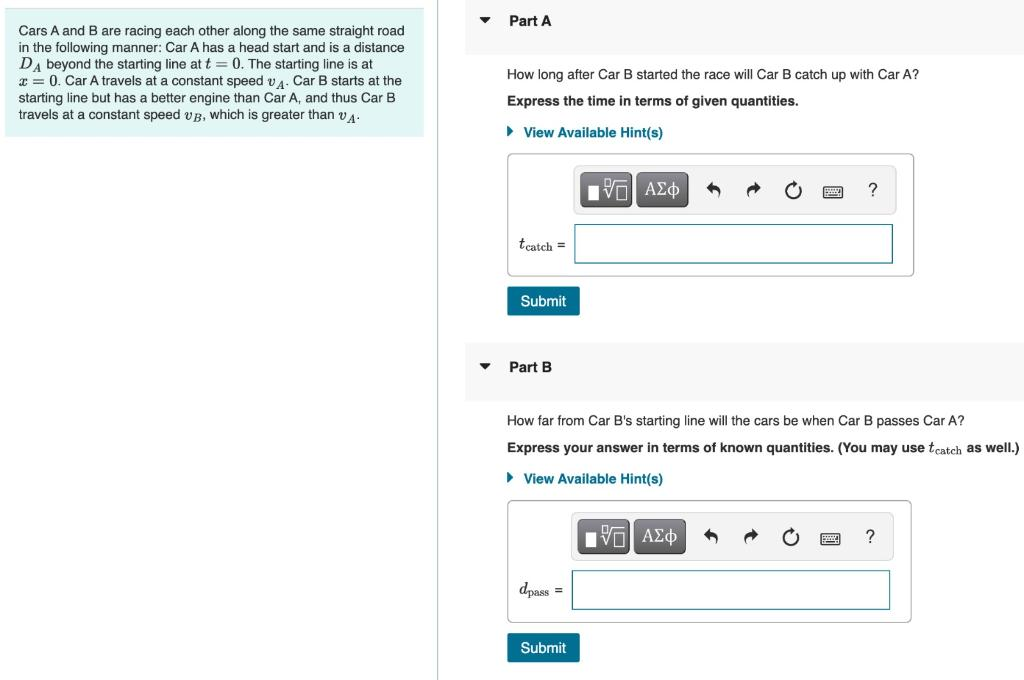# Question Solved1 Answer Cars A and B are racing each other along the same straight road in the following manner: Car A has a head start and is a distance DA beyond the starting line at t = 0. The starting line is at x = 0. Car A travels at a constant speed A. Car B starts at the starting line but has a better engine than Car A, and thus Car B travels at a constant speed up, which Cars A and B are racing each other along the same straight road in the following manner: Car A has a head start and is a distance DA beyond the starting line at t = 0. The starting line is at x = 0. Car A travels at a constant speed A. Car B starts at the starting line but has a better engine than Car A, and thus Car B travels at a constant speed up, which is greater than v Part A How long after Car started the race will Car B catch up with Car A? Express the time in terms of given quantities. ▸ View Available Hint(s) D IVE ΑΣΦ ? tcatch = Submit Part B How far from Car B's starting line will the cars be when Car I passes Car A? Express your answer in terms of known quantities. (You may use tcatch as well.) ► View Available Hint(s) [ΨΕΙ ΑΣΦ ? dpass = SubmitTranscribed Image Text: Cars A and B are racing each other along the same straight road in the following manner: Car A has a head start and is a distance DA beyond the starting line at t = 0. The starting line is at x = 0. Car A travels at a constant speed A. Car B starts at the starting line but has a better engine than Car A, and thus Car B travels at a constant speed up, which is greater than v Part A How long after Car started the race will Car B catch up with Car A? Express the time in terms of given quantities. ▸ View Available Hint(s) D IVE ΑΣΦ ? tcatch = Submit Part B How far from Car B's starting line will the cars be when Car I passes Car A? Express your answer in terms of known quantities. (You may use tcatch as well.) ► View Available Hint(s) [ΨΕΙ ΑΣΦ ? dpass = Submit
More
Transcribed Image Text: Cars A and B are racing each other along the same straight road in the following manner: Car A has a head start and is a distance DA beyond the starting line at t = 0. The starting line is at x = 0. Car A travels at a constant speed A. Car B starts at the starting line but has a better engine than Car A, and thus Car B travels at a constant speed up, which is greater than v Part A How long after Car started the race will Car B catch up with Car A? Express the time in terms of given quantities. ▸ View Available Hint(s) D IVE ΑΣΦ ? tcatch = Submit Part B How far from Car B's starting line will the cars be when Car I passes Car A? Express your answer in terms of known quantities. (You may use tcatch as well.) ► View Available Hint(s) [ΨΕΙ ΑΣΦ ? dpass = Submit